# Possible to solve for the initial speed of a projectile?

• silkms

#### silkms

Is it possible to solve for the initial speed of projectile hitting a target given that we know the direction (vector) that it is released at and the point on a the surface that it is hitting? If so how would you go about finding an equation to solve for this?

I realize that this is a relatively easy problem to solve when gravity is always aiming in the same direction, but in the particular case I am trying to solve, gravity is varying as it flies through the air. Basically think of a projectile being hit around a mini planet (see the image below), where the force of gravity on the projectile is,

g = Gconst * mmass planet / rdist2

The trajectory trail in the image below is generated from simulating the projectile's flight and adding or removing initial speed to bring it closer to the target position based on the "final" position. This would obviously be done more efficiently by using an equation that would allow me to solve for the initial speed given the initial direction and final position.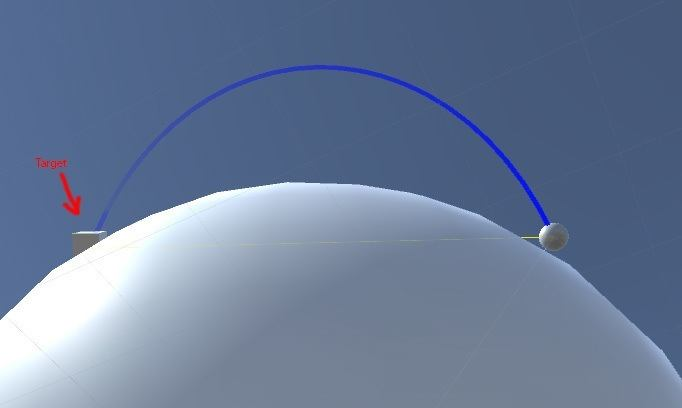Any help solving this is very much appreciated.

If so how would you go about finding an equation to solve for this?
The trajectory is an ellipse. And the speed along it obeys Kepplers Laws.

Nidum, A.T. Thanks! Looking into both.

You could step wise solve it by keeping gravity constant in chunks.

First we consider that the path will be a section of an elliptical orbit, with the apogee at the top of the arc. This puts the perigee 180 degrees or pi radians away (and under the surface of the Earth.) To work this problem out, you have to work out the particulars of this orbit.

First we find the eccentricity. For this, we use the equation:

(1)## \tan f = \frac{e \sin q}{1+ e \cos q}##

where f is the angle from the horizontal our projectile is launched, e is the eccentricity, and q the angle from perigee to the launch point.

If we work in radians we can get q by

(2)## q = \pi- \frac{d}{2R_p}##
where d is the distance between launch and landing points as measured along the surface of the planet and Rp is the radius of the planet.

With q and f we can solve for e with equation 1.

Now we work out the semi-major axis of the orbit using
(3)## R_p= \frac{a (1-e^2)}{1+e \cos q}##

And solving for 'a', the semi-major axis.

The total orbital energy of the projectile is:

(4) ##E = -\frac{GMm}{2a}##

Where G is the gravitational constant, M the mass of the planet and m the mass of the projectile ( don't worry that we don't have a value for m, that will take care of itself later.)You will note that this is a negative value. This is due to how zero gravitational potential is assigned in orbital mechanics.

Another way to find the total energy is to add the kinetic and gravitational potential energies of the projectile at the moment of launch together, or

(5)##E = \frac{mv^2}{2}-\frac{GMm}{R_p}##

Equating equations 4 and 5 gives us:

(6)## -\frac{GMm}{2a}=\frac{mv^2}{2}-\frac{GMm}{R_p}##

The 'm's cancel out (which is why we don't have to worry about the mass of the projectile*) and we can solve for 'v', the speed of the projectile at launch.

* We are assuming here that m is very small compared to M. If this is not the case, then this has to be accounted for in the calculations.

Last edited:
Janus, Thank you for your very clear response. It has been very helpful!

I've followed your reasoning and tried applying it, but am not getting the correct result.

After looking at it a bit more, I don't believe that we can use the radius of the planet (Rp) as the distance from the center of the ellipse. If I'm not mistaken the center of the ellipse will only be at the center of the sphere in very limited circumstances.

That being said, I have tried finding the center of the ellipse (which would allow us to correctly find all the missing pieces), but that has eluded me so far.

Janus, Thank you for your very clear response. It has been very helpful!

I've followed your reasoning and tried applying it, but am not getting the correct result.

After looking at it a bit more, I don't believe that we can use the radius of the planet (Rp) as the distance from the center of the ellipse. If I'm not mistaken the center of the ellipse will only be at the center of the sphere in very limited circumstances.

That being said, I have tried finding the center of the ellipse (which would allow us to correctly find all the missing pieces), but that has eluded me so far.

The center of the ellipse is not going to be at the center of the planet, but one of it foci will be. The following image illustrate the parameters of the trajectory. Note that the center of the planet and the focus of the orbit are considered the same point. This is one of the things we can treat as true if the mass of our projectile is very small compared to that of the planet. Once the projectile mass becomes significant with respect to the planet, we have to account for the fact that this can no longer be considered the case.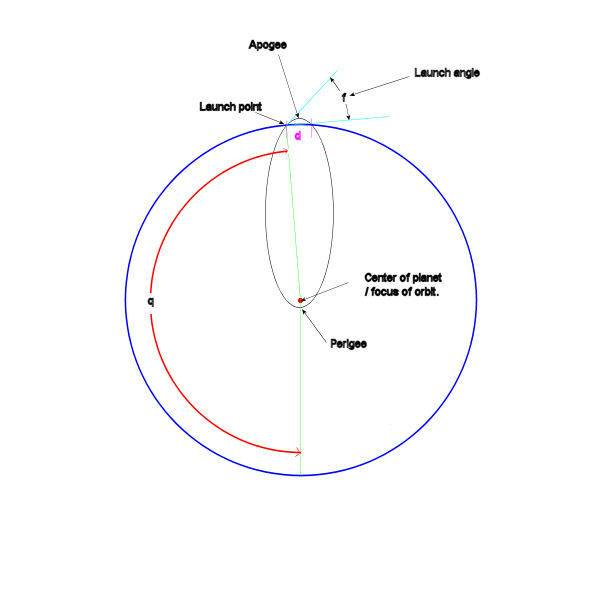As an example, let's work out the launch speed for a travel distance of 100 km and a launch angle(f) of 1 radian ( 57.3 degrees), using the Earth as our planet.
The radius of the Earth is 6378 km, so using eq. 2, we get 3.1338 rad for q.
Using this value and the above given launch angle, we solve for e using eq. 1, and get an answer of 0.995022
Using equation 3 we solve for a, the semi-major axis of the orbit. (the reason we use Rp in this equation is because this is the distance the projectile is from the focus of the orbit when it is launched and when it is an angle of q from the perigee)
Solving for a gives us 3216.3595 km, which we need to convert to 3216359.5 meters for the next step

Finally using the total energy equations (also being sure to convert Rp to meters) and 3.987e14 m3/s2 for GM,

we can solve for v and get an answer of 1031.3 m/s.

If we use this velocity and the same launch angle of 1 radian, we can work out how far the projectile would travel if we assumed a constant gravity always pointing in the same direction. The vertical component of the launch velocity would be equal to the sine of the launch angle times the launch velocity or 867.8 m/s. This means that the projectile would be in the air for 2 x 867.8/9.8 or 177 sec. The horizontal velocity of the projectile is equal to the cosine of the launch angle times the launch velocity or 557.2 m/s, and would travel 557.2 m/s x 177s =98626.8 m or 98.6268 km in horizontal distance. This is just a bit shorter than the 100 km you get with the same launch speed and angle while taking the curve of the Earth and the change in gravity into account, and is just what we would expect.

Janus, thank you for the second breakdown. Went back and found the error in my initial setup and it is now working. Very much appreciated!

Last edited:
New wrinkle.

If the position on the ellipse of the second point becomes arbitrary (i.e. not necessarily on the surface of the planet). How could you go about solving this?

I have attempted to find q (the angle to the periapsis) with the new scenario but not having much luck there. Any ideas for finding this or the semi major axis directly?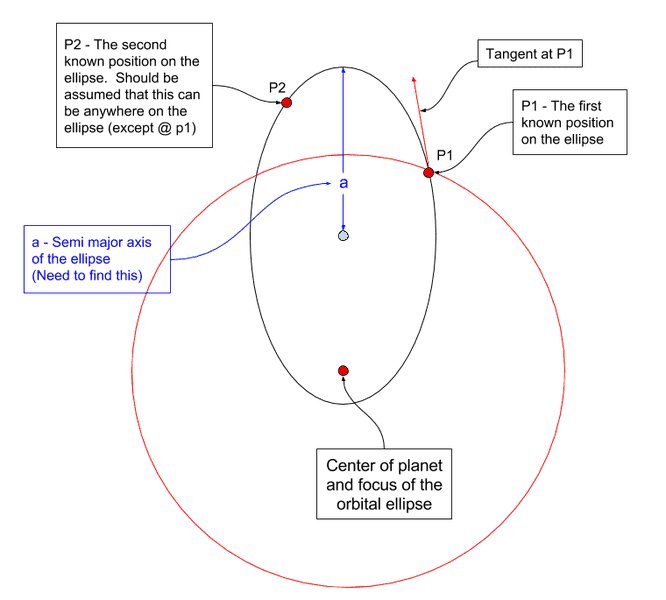Sorry about taking so long getting back to you on this, but it took some head scratching on my part to get an answer.
Here's what I ended up with:

I started with defining the positions of P1 and P2 as their radial distances from the center of the planet and their angles from perigee as shown here: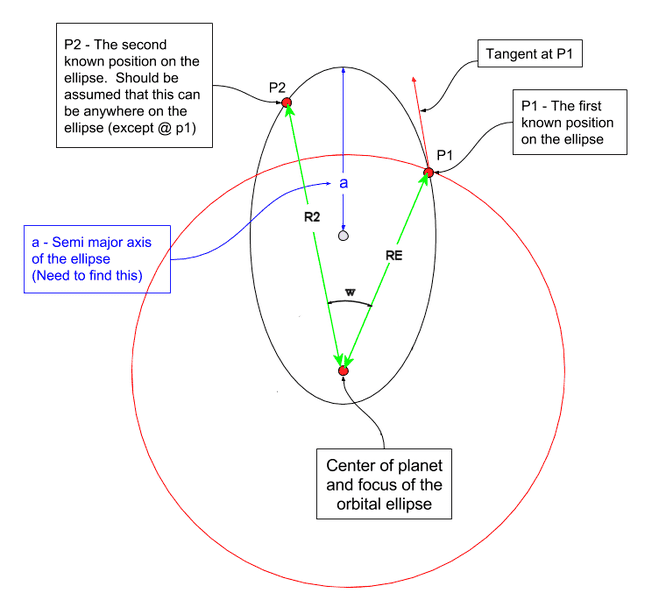RE is the radial distance of P1 and R2 is the radial distance of P2. If q is the angle between perigee and the P1 radial, and w is the angle between the P1 and P2 radials, then q+w is the angle between perigee and the P2 radial.

With this information and re-arranging eq 3 from post #6 to solve for a, we can set up the following equality:

$$\frac{R_E (1+e \cos q)}{1-e^2}= \frac{R_2 (1+e \cos (q+w))}{1-e^2}$$

we solve for e and equate this solution to eq 1 from the same post.

Then, with the help of some trig identities, we finally end up with a solution for q using just the factors we know.
$$\tan q = \frac{2 R_E - R_2 \cos w - R_2}{R_2 \sin w + \cot f (R_2-R_E)}$$
Rather then post the Latex of this solution, I attached an image of it written out. I hope this helps.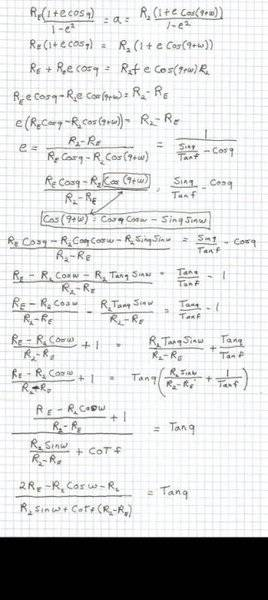Janus, works great. Thanks again!